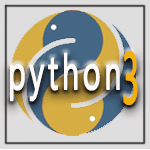﻿ Python Arrow Module: Parse a string representing a time according to a format - w3resource# Python Arrow Module: Parse a string representing a time according to a format

## Arrow Module: Exercise-23 with Solution

Write a Python program to parse a string representing a time according to a format.

Sample Solution:

Python Code:

``````import arrow
a = arrow.utcnow()
print("Current datetime:")
print(a)
print("\ntime.struct_time, in the current timezone:")
print(arrow.utcnow().timetuple())
``````

Sample Output:

```Current datetime:
2019-06-04T06:16:14.385600+00:00

time.struct_time, in the current timezone:
time.struct_time(tm_year=2019, tm_mon=6, tm_mday=4, tm_hour=6, tm_min=16, tm_sec=14, tm_wday=1, tm_yday=155, tm_isdst=0)
```

Python Code Editor:

Have another way to solve this solution? Contribute your code (and comments) through Disqus.

What is the difficulty level of this exercise?

﻿